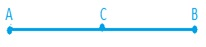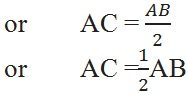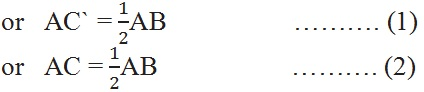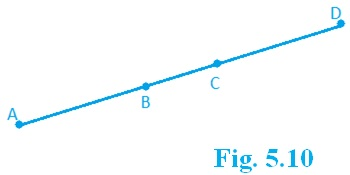Study Materials: ncert solutions

Our ncert solutions for Exercise 5.1 Class 9 maths 5. Introduction To Euclid’s Geometry - ncert solutions - Toppers Study is the best material for English Medium students cbse board and other state boards students.

Solutions ⇒ Class 9th ⇒ Mathematics ⇒ 5. Introduction To Euclid’s Geometry

# Exercise 5.1 Class 9 maths 5. Introduction To Euclid’s Geometry - ncert solutions - Toppers Study

Topper Study classes prepares ncert solutions on practical base problems and comes out with the best result that helps the students and teachers as well as tutors and so many ecademic coaching classes that they need in practical life. Our ncert solutions for Exercise 5.1 Class 9 maths 5. Introduction To Euclid’s Geometry - ncert solutions - Toppers Study is the best material for English Medium students cbse board and other state boards students.

## Exercise 5.1 Class 9 maths 5. Introduction To Euclid’s Geometry - ncert solutions - Toppers Study

CBSE board students who preparing for class 9 ncert solutions maths and Mathematics solved exercise chapter 5. Introduction To Euclid’s Geometry available and this helps in upcoming exams 2023-2024.

### You can Find Mathematics solution Class 9 Chapter 5. Introduction To Euclid’s Geometry

• All Chapter review quick revision notes for chapter 5. Introduction To Euclid’s Geometry Class 9
• NCERT Solutions And Textual questions Answers Class 9 Mathematics
• Extra NCERT Book questions Answers Class 9 Mathematics
• Importatnt key points with additional Assignment and questions bank solved.

NCERT Solutions do not only help you to cover your syllabus but also will give to textual support in exams 2023-2024 to complete exercise 5.1 maths class 9 chapter 5 in english medium. So revise and practice these all cbse study materials like class 9 maths chapter 5.1 in english ncert book. Also ensure to repractice all syllabus within time or before board exams for ncert class 9 maths ex 5.1 in english.

See all solutions for class 9 maths chapter 5 exercise 5 in english medium solved questions with answers.

### Exercise 5.1 class 9 Mathematics Chapter 5. Introduction To Euclid’s Geometry

• Exercise 5.1 Class 9 Maths 5. Introduction To Euclid’s Geometry - Ncert Solutions - Toppers Study
• Class 9 Ncert Solutions
• Solution Chapter 5. Introduction To Euclid’s Geometry Class 9
• Solutions Class 9
• Chapter 5. Introduction To Euclid’s Geometry Exercise 5.1 Class 9

## Exercise 5.1 Class 9 maths 5. Introduction To Euclid’s Geometry - ncert solutions - Toppers Study

Chapter 5. ntroduction to euclid 's geometry

Excercise : 5.1

1. Which of the following statements are true and which are false? Give reasons for your answers.

(i) Only one line can pass through a single point.

(ii) There are an infinite number of lines which pass through two distinct points.

(iii) A terminated line can be produced indefinitely on both the sides.

(iv) If two circles are equal, then their radii are equal.

(v) In Fig. 5.9, if AB = PQ and PQ = XY, then AB = XYSolution :

(i) false, infinite lines can pass through a point.

(ii) false, only single line can pass through two distinct point.

(iii) true, they can be produced both sides indefinitely.

(iv) true, all the circles are drawn from radius.

(v) true, all three lines are equal to each other.

2. Give a definition for each of the following terms. Are there other terms that need to be defined first? What are they, and how might you define them?

(i) parallel lines

(ii) perpendicular lines

(iii) line segment

(v) square

Solution :

(i) the two lines never meet each other and distance between them are equal.

(ii) the two lines stand on each other such that the angle between them is a right angle .

(iii) the lines which has two point.

(iv) the distance between centre and circumference.

(v) the closed figure which has all sides equal.

3. Consider two ‘postulates’ given below:

(i) Given any two distinct points A and B, there exists a third point C which is in between A and B.

(ii) There exist at least three points that are not on the same line.

Do these postulates contain any undefined terms? Are these postulates consistent? Do they follow from Euclid’s postulates? Explain.

Solution :

Yes, this postulate  contain two undefined terms that lines and point.

Yes, this postulates are inconsistent because they deal with two different situation and none of them follow the euclid’s postulate because it is based on axiom .

4. If a point C lies between two points A and B such that AC = BC, then prove that AC = AB. Explain by drawing the figure.

Solution :

Given : AC = BCTo prove : AC = AB

Proof : AC +BC = AB

or         AC + AC = AB

or         2AC = AB5. In Question 4, point C is called a mid-point of line segment AB. Prove that every line segment has one and only one mid-point.

Solution :

C is the mid-point of AB

So,  AC = BC

Let, C` is a point on AB. Which is the mid-point of AB

So, AC` = BC`By eq (1) and (2)

AC`= AC

or  C`= C

So, C and C` is same mid-point mean it is coincident.

6. In Fig. 5.10, if AC = BD, then prove that AB = CD.Solution:

Given: AC = BD

To prove: AB = CD

Proof:  AC = BD                   ……… (1)

Subtracting BC from eq (1)

AC – BC = BD – BC

AB = CD

7. Why is Axiom 5, in the list of Euclid’s axioms, considered a ‘universal truth’? (Note that the question is not about the fifth postulate.)

Solution :

This is true in anything in any part of the world therefore, it is universal.

##### Other Pages of this Chapter: 5. Introduction To Euclid’s Geometry

Important Study materials for classes 06, 07, 08,09,10, 11 and 12. Like CBSE Notes, Notes for Science, Notes for maths, Notes for Social Science, Notes for Accountancy, Notes for Economics, Notes for political Science, Noes for History, Notes For Bussiness Study, Physical Educations, Sample Papers, Test Papers, Mock Test Papers, Support Materials and Books.Mathematics Class - 11th

NCERT Maths book for CBSE Students.

books

## Study Materials List:

##### Solutions ⇒ Class 9th ⇒ Mathematics
1. Number Systems
2. Polynomials
3. Coordinate Geometry
4. Linear Equation In Two Variables
5. Introduction To Euclid’s Geometry
6. Lines and Angles
7. Triangles